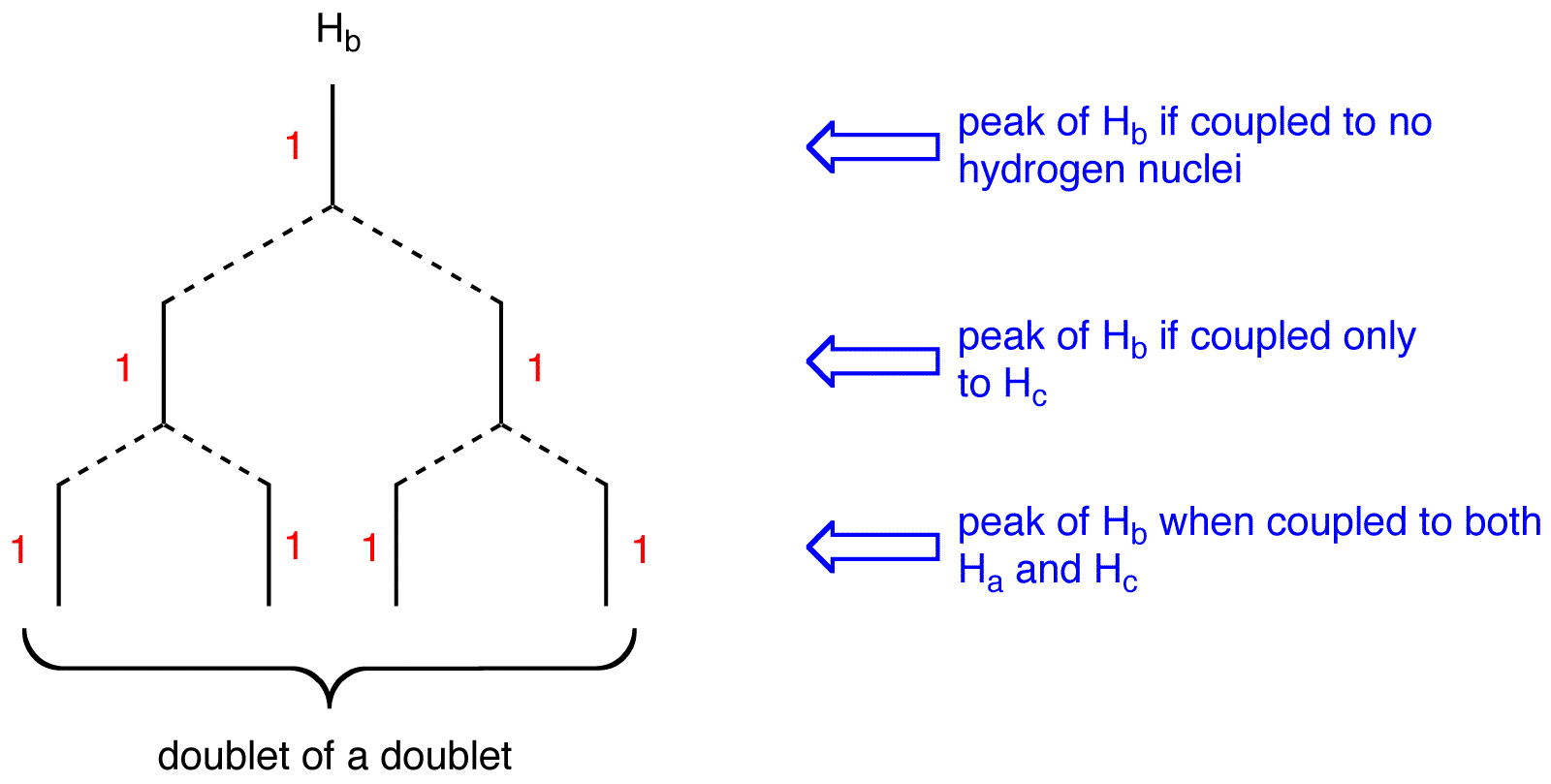# 14.8: The n+1 Rule Applies Only to First-Order Spectra

The (n+1) Rule, an empirical rule used to predict the multiplicity and, in conjunction with Pascal’s triangle, splitting pattern of peaks in 1H and 13C NMR spectra, states that if a given nucleus is coupled (see spin coupling) to n number of nuclei that are equivalent (see equivalent ligands), the multiplicity of the peak is n+1. eg. 1:The three hydrogen nuclei in 1, $$H_a$$, $$H_b$$, and $$H_c$$, are equivalent. Thus, 1H NMR spectrum of 1 $$H_a$$s only one peak. $$H_a$$, $$H_b$$, and $$H_c$$ are coupled to no hydrogen nuclei. Thus, for $$H_a$$, $$H_b$$, and $$H_c$$, n=0; (n+1) = (0+1) = 1. The multiplicity of the peak of $$H_a$$, $$H_b$$, and $$H_c$$ is one. The peak $$H_a$$s one line; it is a singlet. eg. 2:There are two sets of equivalent hydrogen nuclei in 2:

• Set 1: $$H_a$$
• Set 2: $$H_b$$, $$H_c$$

Thus, the 1H NMR spectrum of 2 $$H_a$$s two peaks, one due to $$H_a$$ and the other to $$H_b$$ and $$H_c$$.

The peak of $$H_a$$: There are two vicinal hydrogens to $$H_a$$: $$H_b$$ and $$H_c$$. $$H_b$$ and $$H_c$$ are equivalent to each other but not to $$H_a$$. Thus, for $$H_a$$, n=2; (n+1) = (2+1) = 3. The multiplicity of the peak of $$H_a$$ is three. The peak $$H_a$$s three lines; from the Pascal’s triangle, it is a triplet.

The peak of $$H_b$$ and $$H_c$$: There is only one vicinal hydrogen to $$H_b$$ and $$H_c$$: $$H_a$$. $$H_a$$ is not equivalent to $$H_b$$ and $$H_c$$. Thus, for $$H_b$$ and $$H_c$$, n=1; (n+1) = (1+1) = 2. The multiplicity of the peak of $$H_b$$ and $$H_c$$ is two. The peak $$H_a$$s two lines, from the Pascal’s triangle, it is a doublet.

To determine the multiplicity of a peak of a nucleus coupled to more than one set of equivalent nuclei, apply the (n+1) Rule independently to each other.

eg:There are three set of equivalent hydrogen nuclei in 3:

• Set 1: $$H_a$$
• Set 2: $$H_b$$
• Set 3: $$H_c$$peak of $$H_a$$:multiplicity of the peak of $$H_a = 2 \times 2 = 4$$. To determine the splitting pattern of the peak of $$H_a$$, use the Pascal’s triangle, based on the observation that, for alkenyl hydrogens, $$J_{cis} > J_{gem}$$.The peak of $$H_a$$ is a doublet of a doublet.

peak of $$H_b$$:multiplicity of the peak of $$H_b = 2 \times 2 = 4$$. To determine the splitting pattern of the peak of $$H_b$$, use the Pascal’s triangle, based on the observation that, for alkenyl hydrogens, $$J_{trans} > J_{gem}$$.The peak of $$H_b$$ is a doublet of a doublet.

peak of $$H_c$$:multiplicity of the peak of $$H_c = 2 \times 2 = 4$$. To determine the splitting pattern of the peak of $$H_c$$, use the Pascal’s triangle based on the observation that, for alkenyl hydrogens, $$J_{trans} > J_{cis}$$.The peak of $$H_c$$ is a doublet of a doublet.